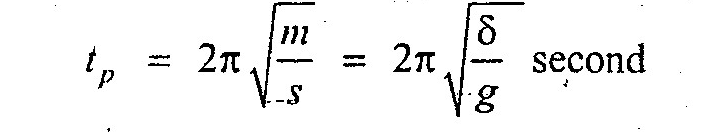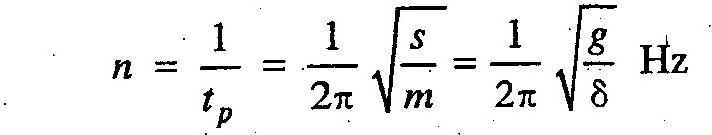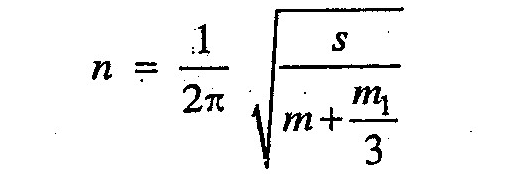### Closely Coiled Helical Spring

Consider a closely coiled helical spring whose one end is fixed and the other end carries a load W=mg, as shown in Fig. 1.34. Let AA be the equilibrium position of the spring after the load is attached. If the spring is stretched upto BB and then released, the load will move up and down with simple harmonic    motion.

For a closely coiled helical spring, periodic time,and frequency of oscillation,where

s = Stiffness of the spring, and
δ = Deflection of the spring.

If the mass of the spring (m1) is also taken into consideration, then frequency of oscillation,